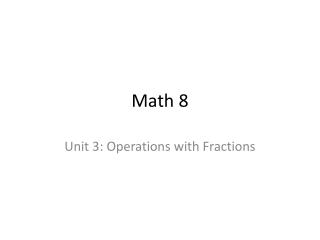DownloadDownload PresentationMath 8

# Math 8

Télécharger la présentation## Math 8

- - - - - - - - - - - - - - - - - - - - - - - - - - - E N D - - - - - - - - - - - - - - - - - - - - - - - - - - -
##### Presentation Transcript

1. Math 8 Unit 3: Operations with Fractions

2. Unit 3: Operations with Fractions What you’ll learn • Estimate the products and quotients of fractions and mixed numbers • Multiply a fraction by a whole number and by a fraction • Divide a fraction by a whole number and by a fraction • Multiply and divide mixed numbers • Use the order of operations with fractions

3. Unit 3: Operations with Fractions Why it’s important: • Used with cooking for measuring • Used with shopping (sales ½ off) • Used with construction for measuring

4. Fraction Review Review Fractions are used to show parts of a whole. Three parts out of four are coloured in. One part out of four is white.

5. Fraction Review Review • The top is called the numerator. • The bottom is called the denominator. • The line is called the fraction bar. numerator fraction bar denominator

6. Fraction Review Review Improper fractions have a numerator larger than the denominator. Mixed fractions are a combination of whole number and fraction. Improper fraction Mixed fraction

7. Fraction Review Review Equivalent fractions – have the same value, they are multiples of each other.

8. Fraction Review Review Find the smallest equivalent fraction. A. B. C. D.

9. Equivalent fraction animation

10. Fraction Review Review To add or subtract fractions they must have the same denominator. Use equivalent fractions to get the same denominator.

11. Fraction Review Make equivalent fractions by doubling the quarter. The denominator must be the same for both fractions. Reduce the fraction to the smallest possible equivalent fraction.

12. Fraction Review Make equivalent fractions by halving the two eighths. The denominator must be the same for both fractions. Reduce the fraction to the smallest possible equivalent fraction.

13. Unit 3: Operations with Fractions 3.1 Using Models To Multiply Fractions and Whole Numbers

14. 3.1 Using Models To Multiply Fractions and Whole Numbers • Focus: Use models to multiply a fraction by a whole number

15. 3.1 Using Models To Multiply Fractions and Whole Numbers • To add fraction you must have the same denominator. 3 8 1 4 = +

16. 3.1 Using Models To Multiply Fractions and Whole Numbers • We change the second fraction into eighths. 3 8 2 8 = +

17. 3.1 Using Models To Multiply Fractions and Whole Numbers • We add the numerators. 3 8 2 8 3+2 8 5 8 = = +

18. 3.1 Using Models To Multiply Fractions and Whole Numbers Remember • Multiplication is accelerated addition • 2 + 2 + 2 = 6 • This can also be done as three groups of two 3 2 x 3 = 6 2

19. 3.1 Using Models To Multiply Fractions and Whole Numbers • Match the addition and multiplication • 3 + 3 + 3 + 3 • 5 + 5 • 2 + 2 + 2 + 2 + 2 • 1 + 1 + 1 • 4 x 3 • 1 x 3 • 2 x 5 • 5 x 2

20. Remember

21. 3.1 Using Models To Multiply Fractions and Whole Numbers • What is 3 times two thirds?

22. 3.1 Using Models To Multiply Fractions and Whole Numbers • What is 3 times two thirds?

23. 3.1 Using Models To Multiply Fractions and Whole Numbers • Write out the following multiplications as additions and draw a diagram.

24. Unit 3: Operations with Fractions 3.2 Using Models to Multiply Fractions

25. 3.2 Using Models to Multiply Fractions • Focus: Use models to multiply fractions

26. Unit 3: Operations with Fractions 3.3 Multiplying Fractions

27. 3.3 Multiplying Fractions • Focus: Develop an algorithm to multiply fractions

28. 3.3 Multiplying Fractions • We have seen how to multiply fractions by whole numbers.

29. 3.3 Multiplying Fractions • Multiply the 5 by 18 Method 1 - reduce at end • Reduce by dividing the numerator and denominator by 6

30. 3.3 Multiplying Fractions • Reduce by dividing 18 and 6 by 6. You must do the same to a numerator and denominator. Method 2 - reduce at start 3 • Multiply the numerators, then the denominators. 1

31. 3.3 Multiplying Fractions • Example

32. 3.3 Multiplying Fractions • Example Split the coloured sections into two parts. 1 1 Keep one of the two parts.

33. 3.3 Multiplying Fractions • Example Divide 5 out of 10 in the numerator and out of 5 in the denominator. 2 3 Divide 2 out of 6 (or 2) in the numerator and out of 2 in the denominator. 1 1

34. Unit 3: Operations with Fractions 3.4 Multiplying Mixed Numbers

35. 3.4 Multiplying Mixed Numbers • Focus: Apply knowledge of multiplying fractions to multiply mixed numbers

36. 3.4 Multiplying Mixed Numbers To multiply mixed fractions, change the fraction into an improper fraction. Remember: Improper fraction Mixed fraction

37. 3.4 Multiplying Mixed Numbers • To change from mixed to improper: new numerator whole number x = denominator + numerator

38. 3.4 Multiplying Mixed Numbers • Convert to improper fractions:

39. 3.4 Multiplying Mixed Numbers • To convert to mixed fractions subtract the denominator from the numerator.

40. 3.4 Multiplying Mixed Numbers

41. 3.4 Multiplying Mixed Numbers

42. 3.4 Multiplying Mixed Numbers